# PyTorch专栏（十七）: 使用PyTorch进行深度学习

### 本文是全系列中第5 / 24篇：Pytorch 专栏【磐创AI 导读】：本篇文章讲解了PyTorch专栏的第五章中在深度学习和NLP中使用Pytorch部分的第一小节使用PyTorch进行深度学习查看关于本专栏的介绍：PyTorch专栏开篇查看本章历史文章，请点击下方蓝色字体进入相应链接阅读。

• 使用Sequence2Sequence网络和注意力进行翻译

## 1.深度学习构建模块：仿射变换, 非线性函数以及目标函数

#### 1.1 仿射变换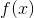PyTorch以及大多数的深度学习框架所做的事情都与传统的线性代数有些不同。它的映射输入是行而不是列。也就是说，下面代码输出的第i行

# Author: Robert Guthrieimport torchimport torch.nn as nnimport torch.nn.functional as Fimport torch.optim as optimtorch.manual_seed(1)lin = nn.Linear(5, 3) # maps from R^5 to R^3, parameters A, b# data is 2x5. A maps from 5 to 3... can we map "data" under A?data = torch.randn(2, 5)print(lin(data)) # yes
• 输出结果：

tensor([[ 0.1755, -0.3268, -0.5069],        [-0.6602, 0.2260, 0.1089]], grad_fn=<AddmmBackward>)

#### 1.2 非线性函数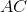是一个矩阵，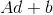是一个向量，可以看出，两个仿射变换的组合还是一个仿射变换。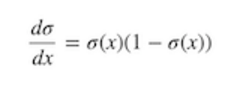σ（x）是默认非线性的，但是通常在实际使用的过程中都会避开它们。这是因为当参数的绝对值增长时，梯度会很快消失。小梯度意味着很难学习。因此大部分人默认选择tanh或者ReLU。

# 在pytorch中，大多数非线性都在torch.函数中（我们将它导入为F）# 请注意，非线性通常没有像仿射图那样的参数。# 也就是说，他们没有在训练期间更新的权重。data = torch.randn(2, 2)print(data)print(F.relu(data))
• 输出结果：

tensor([[-0.5404, -2.2102],        [ 2.1130, -0.0040]])tensor([[0.0000, 0.0000],        [2.1130, 0.0000]])

#### 1.3 Softmax和概率

Softmax(x)也是一个非线性函数，但它的特殊之处在于，它通常是神经网络的最后一个操作。这是因为它接受实数向量，并且返回一个概率分布。它的定义如下。设x为实数向量（正、负，无论什么，没有约束）。然后Softmax(x)的第i个分量是：

# Softmax也在torch.nn.functional中data = torch.randn(5)print(data)print(F.softmax(data, dim=0))print(F.softmax(data, dim=0).sum()) # 总和为1，因为它是一个分布！print(F.log_softmax(data, dim=0)) # theres also log_softmax
• 输出结果：

tensor([ 1.3800, -1.3505, 0.3455, 0.5046, 1.8213])tensor([0.2948, 0.0192, 0.1048, 0.1228, 0.4584])tensor(1.)tensor([-1.2214, -3.9519, -2.2560, -2.0969, -0.7801])

## 3.使用PyTorch创建网络组件

.to(device)方法中的 device 可以是CPU设备torch.device("cpu")或者 CUDA 设备torch.device("cuda:0")

#### 3.1 示例: 基于逻辑回归与词袋模式的文本分类器

[4,0]

[2,2]

[Count(hello),Count(world)]

data = [("me gusta comer en la cafeteria".split(), "SPANISH"),        ("Give it to me".split(), "ENGLISH"),        ("No creo que sea una buena idea".split(), "SPANISH"),        ("No it is not a good idea to get lost at sea".split(), "ENGLISH")]test_data = [("Yo creo que si".split(), "SPANISH"),             ("it is lost on me".split(), "ENGLISH")]# word_to_ix maps each word in the vocab to a unique integer, which will be its# index into the Bag of words vectorword_to_ix = {}for sent, _ in data + test_data:    for word in sent:        if word not in word_to_ix:            word_to_ix[word] = len(word_to_ix)print(word_to_ix)VOCAB_SIZE = len(word_to_ix)NUM_LABELS = 2class BoWClassifier(nn.Module):  # inheriting from nn.Module!    def __init__(self, num_labels, vocab_size):        # calls the init function of nn.Module. Dont get confused by syntax,        # just always do it in an nn.Module        super(BoWClassifier, self).__init__()        # Define the parameters that you will need. In this case, we need A and b,        # the parameters of the affine mapping.        # Torch defines nn.Linear(), which provides the affine map.        # Make sure you understand why the input dimension is vocab_size        # and the output is num_labels!        self.linear = nn.Linear(vocab_size, num_labels)        # NOTE! The non-linearity log softmax does not have parameters! So we don't need        # to worry about that here    def forward(self, bow_vec):        # Pass the input through the linear layer,        # then pass that through log_softmax.        # Many non-linearities and other functions are in torch.nn.functional        return F.log_softmax(self.linear(bow_vec), dim=1)def make_bow_vector(sentence, word_to_ix):    vec = torch.zeros(len(word_to_ix))    for word in sentence:        vec[word_to_ix[word]] += 1    return vec.view(1, -1)def make_target(label, label_to_ix):    return torch.LongTensor([label_to_ix[label]])model = BoWClassifier(NUM_LABELS, VOCAB_SIZE)# 模型知道它的参数。下面的第一个输出是A，第二个输出是b。# 无论何时将组件分配给模块的__init__函数中的类变量，都是使用self.linear = nn.Linear（...）行完成的。# 然后通过PyTorch，你的模块（在本例中为BoWClassifier）将存储nn.Linear参数的知识for param in model.parameters():    print(param)# 要运行模型，请传入BoW矢量# 这里我们不需要训练，所以代码包含在torch.no_grad（）中with torch.no_grad():    sample = data    bow_vector = make_bow_vector(sample, word_to_ix)    log_probs = model(bow_vector)    print(log_probs)
• 输出结果：

{'me': 0, 'gusta': 1, 'comer': 2, 'en': 3, 'la': 4, 'cafeteria': 5, 'Give': 6, 'it': 7, 'to': 8, 'No': 9, 'creo': 10, 'que': 11, 'sea': 12, 'una': 13, 'buena': 14, 'idea': 15, 'is': 16, 'not': 17, 'a': 18, 'good': 19, 'get': 20, 'lost': 21, 'at': 22, 'Yo': 23, 'si': 24, 'on': 25}Parameter containing:tensor([[ 0.1194, 0.0609, -0.1268, 0.1274, 0.1191, 0.1739, -0.1099, -0.0323,         -0.0038, 0.0286, -0.1488, -0.1392, 0.1067, -0.0460, 0.0958, 0.0112,          0.0644, 0.0431, 0.0713, 0.0972, -0.1816, 0.0987, -0.1379, -0.1480,          0.0119, -0.0334],        [ 0.1152, -0.1136, -0.1743, 0.1427, -0.0291, 0.1103, 0.0630, -0.1471,          0.0394, 0.0471, -0.1313, -0.0931, 0.0669, 0.0351, -0.0834, -0.0594,          0.1796, -0.0363, 0.1106, 0.0849, -0.1268, -0.1668, 0.1882, 0.0102,          0.1344, 0.0406]], requires_grad=True)Parameter containing:tensor([0.0631, 0.1465], requires_grad=True)tensor([[-0.5378, -0.8771]])

label_to_ix = {"SPANISH": 0, "ENGLISH": 1}

# 在我们训练之前运行测试数据，只是为了看到之前-之后with torch.no_grad():    for instance, label in test_data:        bow_vec = make_bow_vector(instance, word_to_ix)        log_probs = model(bow_vec)        print(log_probs)# 打印与“creo”对应的矩阵列print(next(model.parameters())[:, word_to_ix["creo"]])loss_function = nn.NLLLoss()optimizer = optim.SGD(model.parameters(), lr=0.1)# 通常，您希望多次传递训练数据.# 100比实际数据集大得多，但真实数据集有两个以上的实例。# 通常，在5到30个epochs之间是合理的。for epoch in range(100):    for instance, label in data:        # 步骤1：请记住，PyTorch会累积梯度。        # We need to clear them out before each instance        model.zero_grad()        # 步骤2：制作我们的BOW向量，并且我们必须将目标作为整数包装在Tensor中。        # 例如，如果目标是SPANISH，那么我们包装整数0.        # 然后，loss函数知道对数概率的第0个元素是对应于SPANISH的对数概率        bow_vec = make_bow_vector(instance, word_to_ix)        target = make_target(label, label_to_ix)        # 步骤3：运行我们的前向传递.        log_probs = model(bow_vec)        # 步骤4：通过调用optimizer.step()来计算损失，梯度和更新参数        loss = loss_function(log_probs, target)        loss.backward()        optimizer.step()with torch.no_grad():    for instance, label in test_data:        bow_vec = make_bow_vector(instance, word_to_ix)        log_probs = model(bow_vec)        print(log_probs)# 对应西班牙语的指数上升，英语下降！print(next(model.parameters())[:, word_to_ix["creo"]])
• 输出结果：

tensor([[-0.9297, -0.5020]])tensor([[-0.6388, -0.7506]])tensor([-0.1488, -0.1313], grad_fn=<SelectBackward>)tensor([[-0.2093, -1.6669]])tensor([[-2.5330, -0.0828]])tensor([ 0.2803, -0.5605], grad_fn=<SelectBackward>)

PyTorch 中文版官方视频教程-60分钟快速入门：

https://www.bilibili.com/video/av66421076/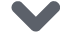下方点击 |   | 了解更多